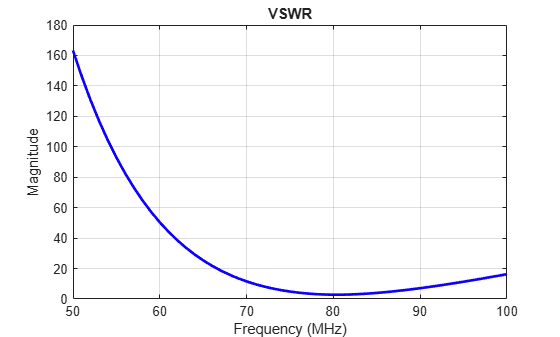# vswr

Voltage standing wave ratio of antenna

## Syntax

``vswr(antenna,frequency,z0)``
``vswrant = vswr(antenna,frequency,z0)``

## Description

example

````vswr(antenna,frequency,z0)` calculates and plots the voltage standing wave ratio of an antenna, over specified frequency range, and given reference impedance, `z0`.```

example

````vswrant = vswr(antenna,frequency,z0)` returns the vswr of the antenna.```

## Examples

collapse all

Plot vswr (voltage standing wave ratio) of a circular loop antenna.

```h = loopCircular; vswr(h,50e6:1e6:100e6,50)```Calculate vswr (voltage standing wave ratio) of a helix antenna.

```h = helix; hvswr = vswr(h,2e9:1e9:4e9,50)```
```hvswr = 1×3 3.5730 6.7043 3.3598 ```

## Input Arguments

collapse all

Antenna object, specified as a scalar handle.

Frequency range used to calculate VSWR, specified as a vector in Hz. The minimum value of frequency must be 1 kHz.

Example: 50e6:1e6:100e6

Data Types: `double`

Reference impedance, specified as a scalar in ohms.

## Output Arguments

collapse all

Voltage standing wave ratio, returned as a vector in dB.